# McGraw Hill Math Grade 1 Chapter 11 Lesson 13 Answer Key Describing Number of Equal Parts

All the solutions provided in McGraw Hill Math Grade 1 Answer Key PDF Chapter 11 Lesson 13 Describing Number of Equal Parts as per the latest syllabus guidelines.

## McGraw-Hill Math Grade 1 Answer Key Chapter 11 Lesson 13 Describing Number of Equal Parts

Describe

Question 1.
Color 1 of 4 equal parts.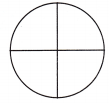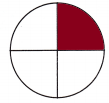Explanation:
1 out of 4 parts is colored.
one fourth of the circle is colored.

Question 2.
Color 3 of 4 equal parts.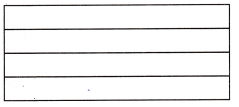Explanation:
The rectangle is divided into 4 equal parts.
3 out of 4 parts are colored.
three fourths are colored.

Question 3.
Color 1 of 2 equal parts.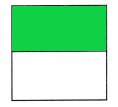Explanation:
The square is divided into 2 equal parts.
1 out of 2 parts are colored
so, half of the square is colored.

Question 4.
Color 4 of 4 equal parts.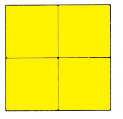Explanation:
The square is divided into 4 equal parts
4 out of 4 parts are colored.

Question 5.
Look at the squares in questions 3 and 4. Which has smaller parts? Tell why.